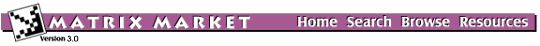# Browse the NEP Collection

Sets:
Generators:
• MATPDE Partial differential equations
• MATRAN Numerical linear algebra
• MVMBWM Chemical engineering
• MVMGRC Numerical linear algebra
• MVMISG Material science
• MVMMCD Computational fluid dynamics
• MVMODE Ordinary differential equations
• MVMRWK Probability theory and its applications
• MVMTLS Aeroelasticity